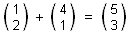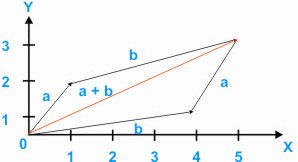HOME MATH DICTIONARY DOWNLOAD FEEDBACK DISCLAIMER
 Question: What is Vector Addition ? Answer: Two vectors are added by adding their corresponding components. For example,When these vectors are represented geometrically as the displacements a and b, their addition is equivalent to following, one displacement by other, and can be represented by arrows as shown. The diagram also shows that the operation of vector addition is commutative, since a + b = b + a.